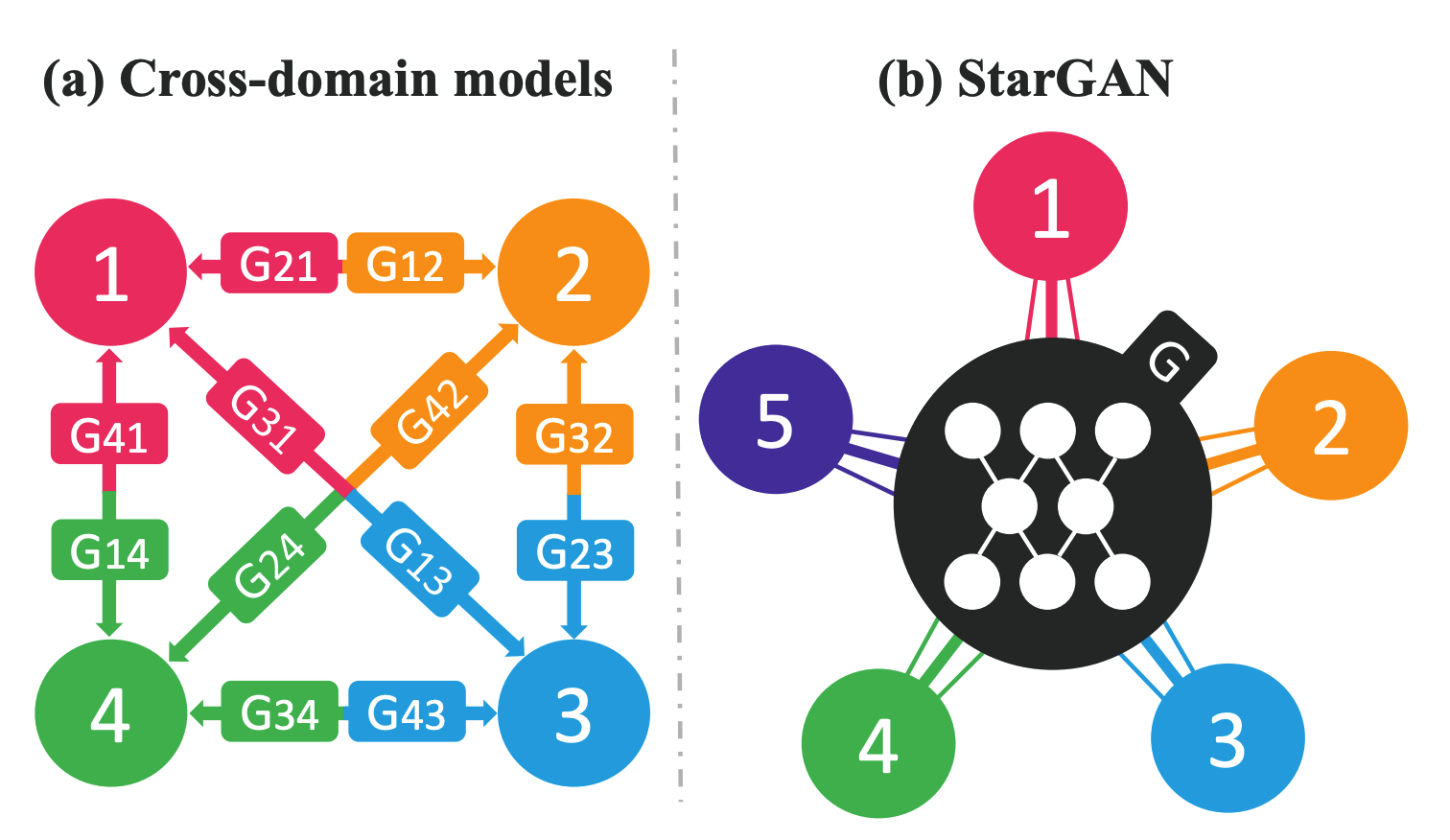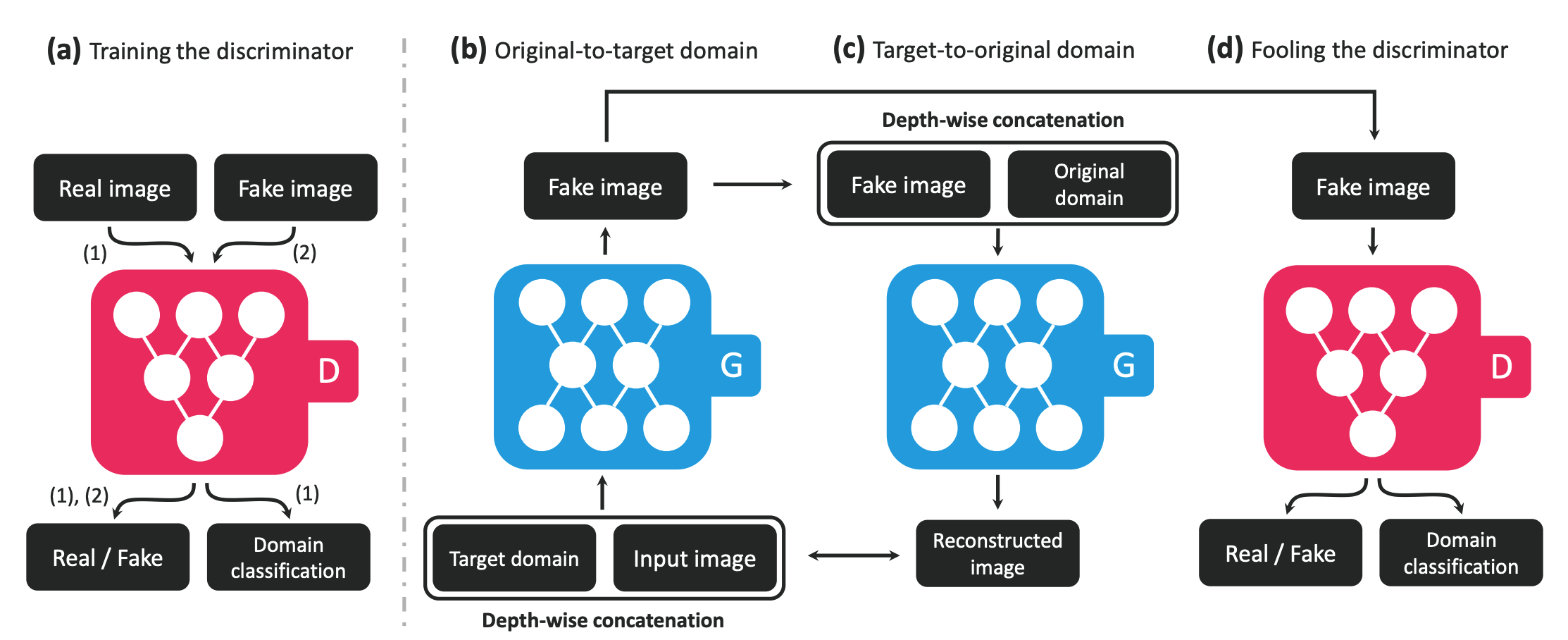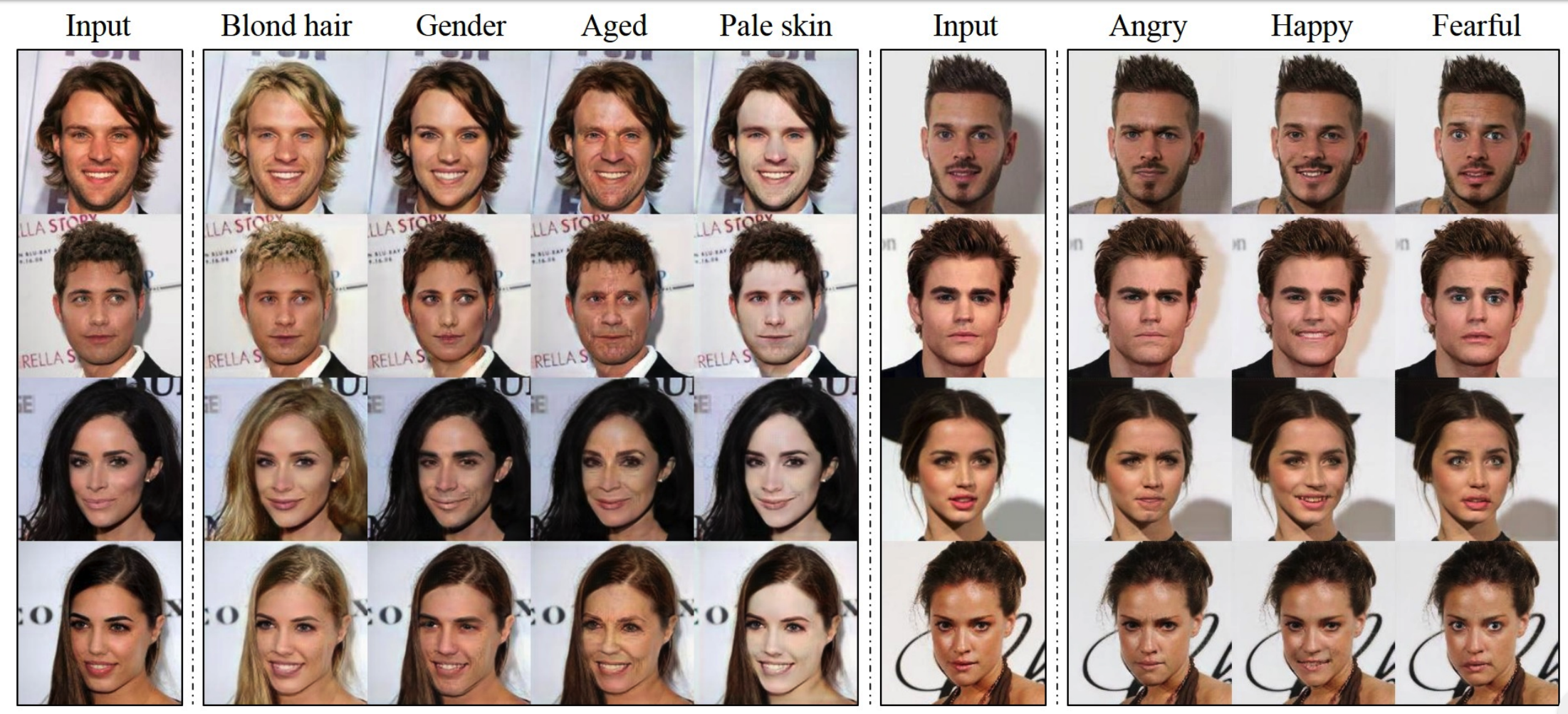# StarGAN: Unified Generative Adversarial Networks for Multi-Domain Image-to-Image Translation

## WHY?CycleGAN has been used in image-to-image translation effectively. However, handling more than two domains was difficult. This paper StarGAN to handle multiple domains with a single model.

## WHAT?

StarGAN can be considered as an domain conditioned version of CycleGAN.The discriminator of StarGAN not only classify real and fake, but also domain labels. The loss function of StarGAN consists of three terms: adversarial loss, domain classification loss, and reconstruction loss.

\mathcal{L}_{adv} = \mathbb{E}_x[\log D_{src}(x)] + \mathbb{E}_{x,c}[\log(1-D_{src}(G(x,c)))]\\
\mathcal{L}_{cls}^r = \mathbb{E}_{x, c'}[-log D_{cls}(c'|x)]\\
\mathcal{L}_{cls}^f = \mathbb{E}_{x, c}[-log D_{cls}(c|G(x, c))]\\
\mathcal{L}_{rec} = \mathbb{E}_{x, c, c'}[\|x - G(G(x, c), c')\|_1]\\
\mathcal{L}_G = \mathcal{L}_{adv} + \lambda_{cls}\mathcal{L}_{cls}^f + \lambda_{rec}\mathcal{L}_{rec}

\lambda_{cls} = 1, \lambda_{rec} = 10$\lambda_{cls} = 1, \lambda_{rec} = 10$ in all of the experiment. To enable StarGAN in multiple domain in datasets with different label information, mask vector is introduced as conditional information.

\tilde{c} = [c_1,...,c_n, m]

Model architecture is adopted from CycleGAN. WGAN-GP objective is used as loss function.

## So?StarGAN successfully generated images conditioned on labels from differnt domains with a single model.

## Critic

I think the key point in StarGAN is effective conditioning of labels. Recent methods from PGGAN or BigGAN or AdaIN from SB-GAN seem to be effective in this setting.

Powered by aiden